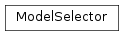# mvpa2.clfs.model_selector.ModelSelector¶class `mvpa2.clfs.model_selector.``ModelSelector`(parametric_model, dataset)

Model selection facility.

Select a model among multiple models (i.e., a parametric model, parametrized by a set of hyperparamenters).

Methods

 `max_log_marginal_likelihood`(hyp_initial_guess) Set up the optimization problem in order to maximize the log_marginal_likelihood. `solve`([problem]) Solve the maximization problem, check outcome and collect results.

TODO:

Methods

 `max_log_marginal_likelihood`(hyp_initial_guess) Set up the optimization problem in order to maximize the log_marginal_likelihood. `solve`([problem]) Solve the maximization problem, check outcome and collect results.
`max_log_marginal_likelihood`(hyp_initial_guess, maxiter=1, optimization_algorithm='scipy_cg', ftol=0.001, fixedHypers=None, use_gradient=False, logscale=False)

Set up the optimization problem in order to maximize the log_marginal_likelihood.

Parameters: parametric_model : Classifier the actual parameteric model to be optimized. hyp_initial_guess : numpy.ndarray set of hyperparameters’ initial values where to start optimization. optimization_algorithm : string actual name of the optimization algorithm. See http://scipy.org/scipy/scikits/wiki/NLP for a comprehensive/updated list of available NLP solvers. (Defaults to ‘ralg’) ftol : float threshold for the stopping criterion of the solver, which is mapped in OpenOpt NLP.ftol (Defaults to 1.0e-3) fixedHypers : numpy.ndarray (boolean array) boolean vector of the same size of hyp_initial_guess; ‘False’ means that the corresponding hyperparameter must be kept fixed (so not optimized). (Defaults to None, which during means all True)

Notes

The maximization of log_marginal_likelihood is a non-linear optimization problem (NLP). This fact is confirmed by Dmitrey, author of OpenOpt.

`solve`(problem=None)

Solve the maximization problem, check outcome and collect results.